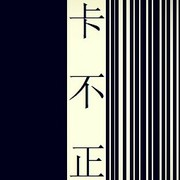{start_of_chorus} I'm only on[C]e cal[G]l awa[Am]y I'll be there to sav[F]e th[C]e da[G]y Superman got n[C]othin[G]g on m[Am]e I'm only o[F]ne cal[G]l awa[C]y {end_of_chorus} [Am] Call me, b[G]aby, if you ne[C]ed a frien[F]d [Am] I just w[G]anna give you lov[C]e C'm[F]on, c'mon, c'm[Am]on Reaching o[G]ut to you, so t[C]ake a cha[F]nce No ma[F]tter where you go You k[G]now you're not alone {start_of_chorus} I'm only on[C]e cal[G]l awa[Am]y I'll be there to sav[F]e th[C]e da[G]y Superman got n[C]othin[G]g on m[Am]e I'm only o[F]ne cal[G]l awa[C]y {end_of_chorus} [Am] Come alo[G]ng with me and d[C]on't be scare[F]d [Am] I just w[G]anna set you fre[C]e C'm[F]on, c'mon, c'm[Am]on You and m[G]e can make it [C]anywh[F]ere For n[Am]ow, we can st[G]ay here for a whi[C]le   [F] 'Cause you kn[Am]ow, I just w[G]anna see you sm[C]ile    [F] No ma[F]tter where you go You k[G]now you're not alone {start_of_chorus} I'm only on[C]e cal[G]l awa[Am]y I'll be there to sav[F]e th[C]e da[G]y Superman got n[C]othin[G]g on m[Am]e I'm only o[F]ne cal[G]l awa[C]y {end_of_chorus} And when you're w[Am]eak,  [G]I'll be s[C]trong[F] I'm gonna k[Am]eep h[G]olding [C]on    [F] Now don't you w[Am]orry, it w[G]on't be l[C]ong D[F]arling, If fe[Am]el like hope is gone Just r[G]un into my arms {start_of_chorus} I'm only on[C]e cal[G]l awa[Am]y I'll be there to sav[F]e th[C]e da[G]y Superman got n[C]othin[G]g on m[Am]e I'm only o[F]ne, I'm only o[F]ne o[C]ne cal[G]l awa[Am]y I'll be there to sav[F]e th[C]e da[G]y Superman got n[C]othin[G]g on m[Am]e I'm only o[F]ne cal[G]l awa[C]y I'm only o[F]ne cal[G]l awa[C]y {end_of_chorus} {title:One call away} {artist:Charlie Puth} {author:_wc_wc_}_wc_wc_